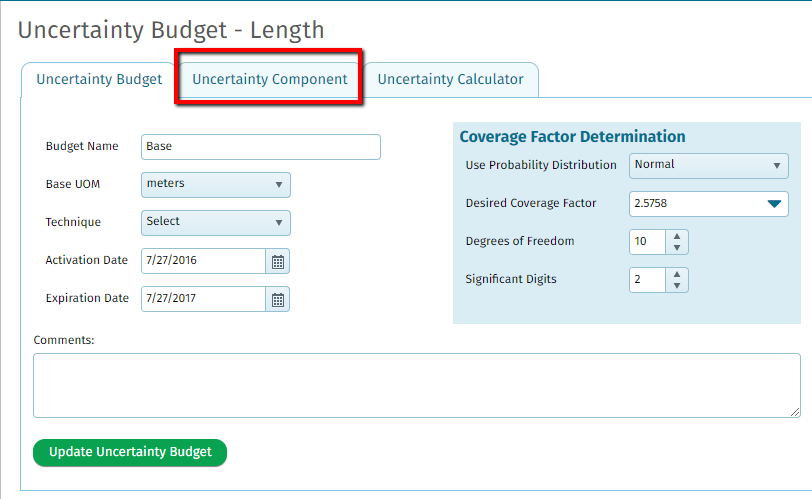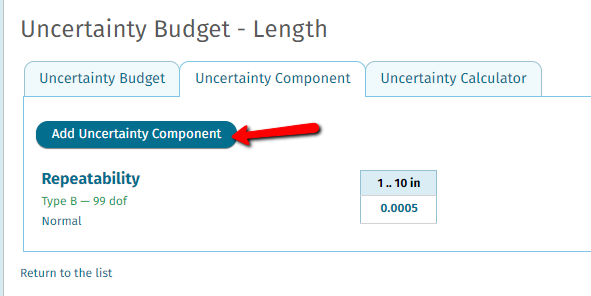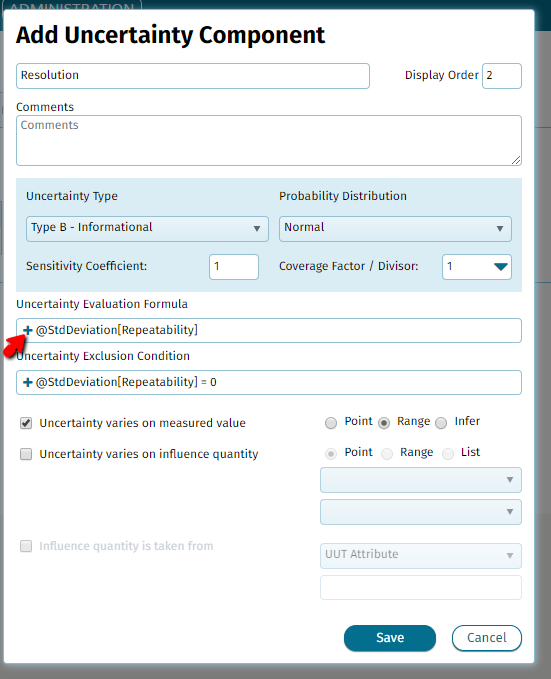*This is a "Feature Updates" article. If you need to get actual instructions, please go to the  "Uncertainty" article.

Users now have the ability to use formula evaluation to configure complex uncertainty budget component calculation scenarios (i.e. some calculations involve more than one measurement parameter or posses conditional logic).Uncertainty budget formulas can use the following operators and variables:

Mathematical operators
Multiply       *
Divide         /
Subtract      -

Math functions
Pow(x, y)  - raise a number "x" to a power "y"
Sqrt(x)      - return square root of "x"

Exclusion condition operators
Less than             <
Greater than        >
Less or equal      <=
Greater or equal >=
Equal                  ==
Not equal           !=
Conditional AND   &&
Conditional OR      ||
Variables
Measurement Point - variable from current measurement point
@Nominal
@Mean
@StdDeviation
@StdError
@ToolAccuracy
@ToolUncertainty
@Error
Different Measurement Point - variable from different measurement point(in square brackets - you can define name of measurement point)
@Nominal[]
@Mean[]
@StdDeviation[]
@StdError[]
@ToolAccuracy[]
@ToolUncertainty[]
@Error[]
Environment Factor - environment factor value
@AirBuoyancy
@AirHumidity
@Altitude
@AmbientTemperature
@BarometricPressure
@EvaporationRate
@LightIntensity
@NoiseLevel
@PhLevel
@WaterConductivity
@WaterTemperature
@WindSpeed
@ZFactor
UUT Attribute - value from unit under test attribute(in square brackets - you can define name of attribute)
@UUT[]
Tool Attribute - value from reference standard attribute(in square brackets - you can define name attrinute)
@Tool[]
Custom Fields - value from custom field(in square brackets - you can define "Field Id" of custom field)
@CustomField[]

Navigate to your uncertainty budgets by clicking 'Administration' from the main menu bar then 'Sites'. Next, click the site name that your budgets are for. Next click 'Uncertainty' from the left side of the screen.Once here, select a budget you would like to modify.Next, click into the 'Uncertainty Component' tab to add or edit the components of this uncertainty budget.Once in the component, input your formula in the 'Uncertainty Evaluation Formula' field. Utilize the '+' to add in variables to the equation.